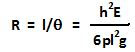Friday, April 10, 2009

Irodov Problem 1.300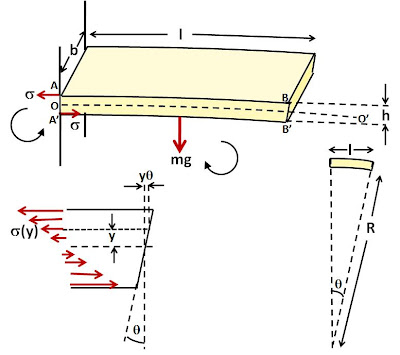Let us first understand whats happening in the problem. The force of gravity acts on the cantilever and induces a torque that aims to turn the cantilever in the clockwise direction. As a result the cantilever bends and curvature is induced into the cantilever. Due to the curvature in the cantilever, the upper surface of the cantilever is stretched while the lower surface is compressed relative to the center line. As seen in the diagram, top surface AB is stretched and is longer than OO' (the center line) and A'B' the bottom surface is smaller than OO'. This distortion results in strain and hence stressin the cantilever. This stress results in a torque in the counter-clockwise direction and balances out the torque due to gravity.

Let the width of the cantilever be b, its density be p. The force of gravity acts at a distance from l/2 from the wall and hence the torque due to gravity will be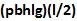.

Suppose that due to the curvature in the cantilever, the end surface bends an angle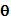as shown in the figure.

Consider an infinitesimally thin section of the cantilever that is at a height y from the center line, and has a thickness of dy. The change in length of this section will be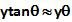as shown in the figure. As a result the strain induced in this section we will be,The stress induced in this section will thus be,The area of this infinitesimally thin section of the cantilever is bdy. The net force due to the stress in this section will thus be. Since the force acts at a distance y from the center, the torque induced by this section will be. The net torque due to the stress in the cantilever can be found as,This torque T due to stress must balance the torque due to gravity for the cantilever to be stable and so we have,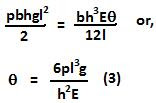Let the radius of curvature seen by the cantilever be R, then as seen from the figure,